# Floor

Rectangular floor of living room has a length 5.4 meters and a circumference 17.2 meters. What is its width?

Result

b =  3.2 m

#### Solution:Leave us a comment of example and its solution (i.e. if it is still somewhat unclear...):

Showing 0 comments:Be the first to comment!## Next similar examples:

1. HotelThe hotel has a p floors each floor has i rooms from which the third are single and the others are double. Represents the number of beds in hotel.
2. PlaygroundRectangular playground is fenced with 38 m long netting. Its width is 7 m. Calculate its length.
3. Cellar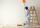Cellar for storing fruit has a rectangular base with sides 14 m and 7 meters. You should paint sidewall to 2 m. How m2 surface must be painted?
4. Arm-legCalculate the length of the base of an isosceles triangle with a circumference 224 cm if the arm length is 68 cm.
5. A rectangleA rectangle has an area of 36 cm2. What could the length and width of rectangle be?
6. Rectangle 45The perimeter of a rectangle is 60cm. If the length of the rectangle is 20cm. a)find the width b)find the area.
7. AlleyAlley measured a meters. At the beginning and end are planted poplar. How many we must plant poplars to get the distance between the poplars 15 meters?
8. Sides of triangle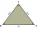Triangle circumference with two identical sides is 117cm. The third side measures 44cm. How many cms do you measure one of the same sides?
9. Circle - simpleThe circumference of a circle is 930 mm. How long in mm is its diameter?
10. SimplifySimplify the following problem and express as a decimal: 5.68-[5-(2.69+5.65-3.89) /0.5]
11. ExpressionSolve for a specified variable: P=a+4b+3c, for a
12. RectangleCalculate perimeter of the rectangle with sides a=2.4 m and b=1.9 m.
13. Addition of Roman numbersAdded together and write as decimal number: LXVII + MLXIV
14. Ratio of perimeters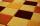Rectangle ABCD has dimensions 3 cm and 4 cm, KLMN rectangle has dimensions 4 cm and 12 cm. Calculate the ratio of the perimeter ABCD and perimeter KLMN.
15. Cuboid box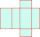Cuboid box have dimensions of 30 cm, 25 cm and 40 cm. Sketch its network and compute surface area of box.
16. RectangleThe rectangle area is 182 dm2, its base is 14 dm. How long is the other side? Calculate its perimeter.
17. Waste paper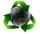Our school attend 1,300 pupils. Each pupil collected plastic bottles or paper. Plastic bottles have collected 930 pupils and 600 pupils old paper. How many students have collected together plastic bottles and waste paper?